## Two long, parallel wires separated by 3.50 cm carry currents in opposite directions. The current in one wire is 1.55 A, and the current in t

Question

Two long, parallel wires separated by 3.50 cm carry currents in opposite directions. The current in one wire is 1.55 A, and the current in the other is 3.15 A. Find the magnitude of the force per unit length that one wire exerts on the other.

in progress 0
4 weeks 2021-08-30T20:43:46+00:00 2 Answers 0 views 0

Therefore,

The magnitude of the force per unit length that one wire exerts on the other is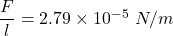Explanation:

Given:

Two long, parallel wires separated by a distance,

d = 3.50 cm = 0.035 meter

Currents,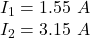To Find:

Magnitude of the force per unit length that one wire exerts on the other,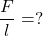Solution:

Magnitude of the force per unit length on each of @ parallel wires seperated by the distance d and carrying currents I₁ and I₂ is given by,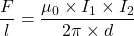where,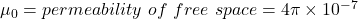Substituting the values we get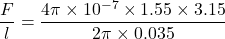Therefore,

The magnitude of the force per unit length that one wire exerts on the other isThe force per unit length is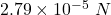.

Explanation:

As depicted in the figure, the current (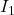) through wire 1 is 1.55 A upwards and the current (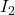) through wire 2 is 3.15 A downwards. The wires are 3.50 cm apart.

From Biot-Savart’s law, we know that the magnetic field (B) due to a current carrying conductor carrying a current I at a distance r from the conductor is given by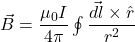According to the figure, the magnetic field (B) at 3.5 cm right to wire 1 due to it can be written as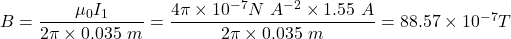andthe direction will be perpendicular to the screen and towards the screen (as depicted by the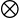symbol in the figure).

Also we know that the force ‘F‘ experience by  a current carrying conductor of length ‘L‘ having current ‘I‘ and placed in a magnetic field ‘B‘ is given by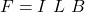So the force per unit length (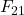) experienced by wire 2 due to the magnetic field due to wire 1 is given by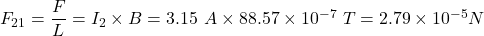and the direction of the force will be right to wire 2.